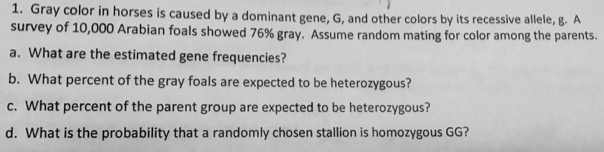# Problem: Gray color in horses iscaused by a dominant gene, G, and other colors by its recessive allele, g. A survey of 10,000 Arabian foals showed 76% gray. Assume random mating for color among parents.A. What are the estimated gene frequencies?B. What percent of the gray foals are expected to be heterozygous?C. What percent of the parent group are expected to be heterozygous?D. What is the probability that a randomly chosen stallion is homozygous GG?

###### FREE Expert Solution

Since it is already assumed that mating is random, and the population is considerably large, if the individuals do not experience genetic drift or mutation of any kind in relation to the gene in question, we can assume that the population follows Hardy-Weinberg equilibrium for the trait. In Hardy-Weinberg equilibrium, it follows the equation, p2 + 2pq + q2 = 1, where each term is for the frequencies of each genotype of a diploid: p2 is the homozygous dominant, 2pq is the heterozygous and q2 is for homozygous recessive.###### Problem Details

Gray color in horses iscaused by a dominant gene, G, and other colors by its recessive allele, g. A survey of 10,000 Arabian foals showed 76% gray. Assume random mating for color among parents.

A. What are the estimated gene frequencies?

B. What percent of the gray foals are expected to be heterozygous?

C. What percent of the parent group are expected to be heterozygous?

D. What is the probability that a randomly chosen stallion is homozygous GG?